Math Gizmos to Learn Multiplication Concepts and Times Tables!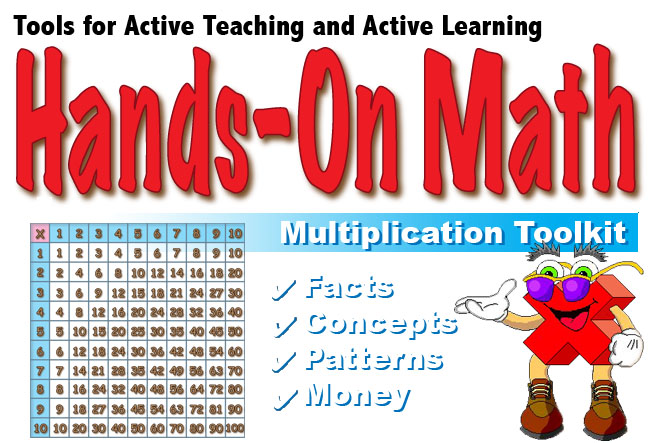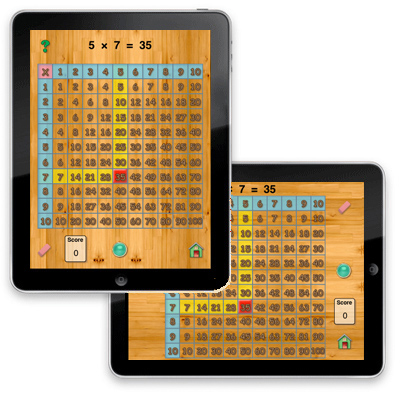The Multiplication Toolkit help students visualize multiplication concepts and is designed to help student improve retention of mulitplication facts. Images representing the multiplication of numbers can be manipulated so that student intuitively understand the meaning of multiplication and increase speed at recalling mulitplication facts. Use the Mulitplication Toolkit to have practice represent products on a classic times table chart. Use the Hundred Chart to mark multiples of numbers from 2 to 10. Practice multiplication facts using flash cards. Illustrated multiple choice quiz provides additional practice. Hands-On Math: Multiplication Toolkit is a collection of instructional devices used to teach mulitiplication concepts and facts.. Base Ten Blocks are one of the best ways to help students place value. Students place Base Ten Blocks on an interactive Playground to represent numbers. Blocks can be regrouped or exchanged by dragging a virtual manipulative from one column to the next. Using Hands-On Math: Multiplication Toolkit students can develop the following important mathematical concepts: Multiplication Concepts Memorization of Times Tables Working with Number Theory Concepts - Prime Numbers Values of U.S. CurrencyWe recommend that you open the Instructor's Guide PDF from your iPad in a new browser page and save the document in your iBooks app.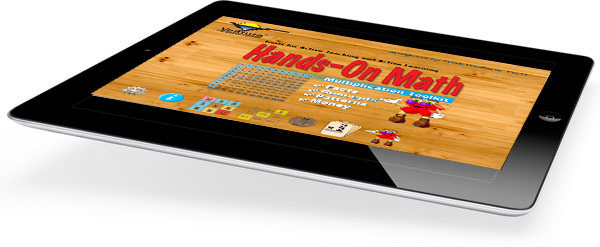Features: Interactive Times Table Chart, Hundred Chart, Flash Cards and Quizzes. Supports Discovery and Directed Teaching Strategies. Problem Solving Activities which Can Be Solved Using Virtual Manipulatives. The Multiplication Toolkit Helps to Build Fundamental Math Concepts. On/Off Options and Controls for Various Features. \$2.99
 Multiplication Toolkit Multiplication Toolkit are used to represent math concepts using a variety of devices such as the classic times table chart and also a hundreds chart. Possible Topics: Meaning of Multiplication Times Tables Retention of Multiplication Facts Two-Step Multiplication Problems. Decimals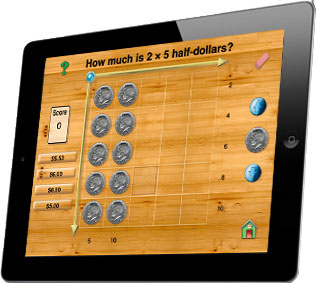A Special Option Just for Australian Educators One of the activities involves finding the value for an array of coins. An option in the settings allows users switch between U.S and Australian currency. Many thanks to the teacher in Australia who sent the coins so we could use the images in this app.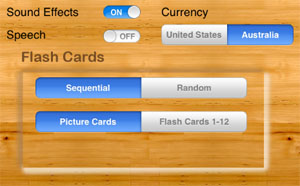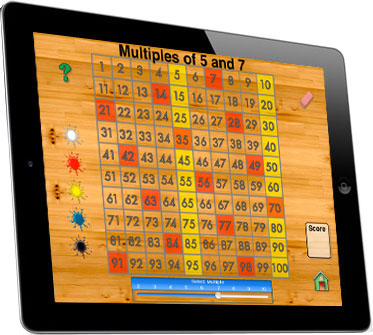Patterns on a Hundreds Chart By manipulating a slider control students can select a multiple from 2 to 10 then by tapping an icon mark the pattern of the multiple on the hundreds chart. As many a four different patterns can be overlayed on the same chart. Explore Multiples of a Specific Number. Practice Skip Counting. Better Understand How to Find a Least Common Multiple. Practice to Improve Skills Activities at a variety of skill levels are also provided. Students are encouraged to document their discoveries in a journal. Here the students are asked to record the problems and answers generated in the quiz to practice reading and writing numbers as well as other important math skills. Perfect for Small Group instruction Environmentally Friendly Learning - (no need to photocopy activity pages for each student) A Student-Centered Approach to Learning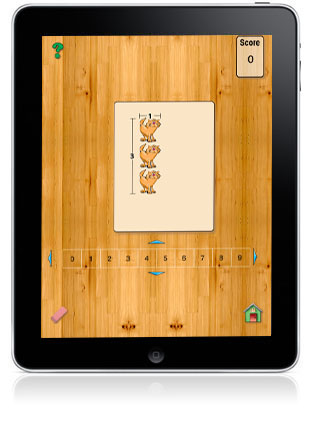Let the Multiplication Toolkit help your students become more proficient at multiplication, an essential skill for success in mathematics.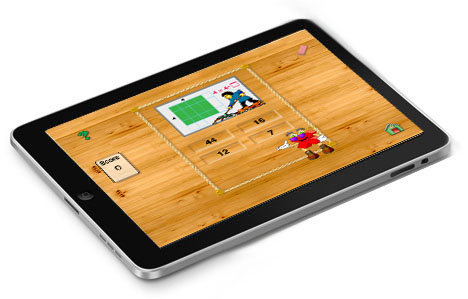Hands-On Math: Multiplication Toolkit is now available at the Apple iTunes Store.